Related Articles
Huffman Coding | Greedy Algo-3
• Difficulty Level : Hard
• Last Updated : 23 Mar, 2021

Huffman coding is a lossless data compression algorithm. The idea is to assign variable-length codes to input characters, lengths of the assigned codes are based on the frequencies of corresponding characters. The most frequent character gets the smallest code and the least frequent character gets the largest code.
The variable-length codes assigned to input characters are Prefix Codes, means the codes (bit sequences) are assigned in such a way that the code assigned to one character is not the prefix of code assigned to any other character. This is how Huffman Coding makes sure that there is no ambiguity when decoding the generated bitstream.
Let us understand prefix codes with a counter example. Let there be four characters a, b, c and d, and their corresponding variable length codes be 00, 01, 0 and 1. This coding leads to ambiguity because code assigned to c is the prefix of codes assigned to a and b. If the compressed bit stream is 0001, the de-compressed output may be “cccd” or “ccb” or “acd” or “ab”.
See this for applications of Huffman Coding.
There are mainly two major parts in Huffman Coding

1. Build a Huffman Tree from input characters.
2. Traverse the Huffman Tree and assign codes to characters.

Steps to build Huffman Tree
Input is an array of unique characters along with their frequency of occurrences and output is Huffman Tree.

1. Create a leaf node for each unique character and build a min heap of all leaf nodes (Min Heap is used as a priority queue. The value of frequency field is used to compare two nodes in min heap. Initially, the least frequent character is at root)
2. Extract two nodes with the minimum frequency from the min heap.

3. Create a new internal node with a frequency equal to the sum of the two nodes frequencies. Make the first extracted node as its left child and the other extracted node as its right child. Add this node to the min heap.
4. Repeat steps#2 and #3 until the heap contains only one node. The remaining node is the root node and the tree is complete.
Let us understand the algorithm with an example:
```character   Frequency
a            5
b           9
c           12
d           13
e           16
f           45```

Step 1. Build a min heap that contains 6 nodes where each node represents root of a tree with single node.
Step 2 Extract two minimum frequency nodes from min heap. Add a new internal node with frequency 5 + 9 = 14.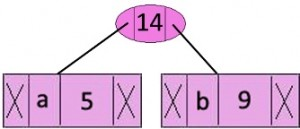Now min heap contains 5 nodes where 4 nodes are roots of trees with single element each, and one heap node is root of tree with 3 elements

```character           Frequency
c               12
d               13
Internal Node         14
e               16
f                45```

Step 3: Extract two minimum frequency nodes from heap. Add a new internal node with frequency 12 + 13 = 25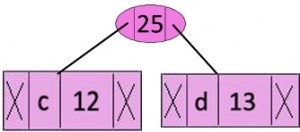Now min heap contains 4 nodes where 2 nodes are roots of trees with single element each, and two heap nodes are root of tree with more than one nodes

```character           Frequency
Internal Node          14
e               16
Internal Node          25
f               45```

Step 4: Extract two minimum frequency nodes. Add a new internal node with frequency 14 + 16 = 30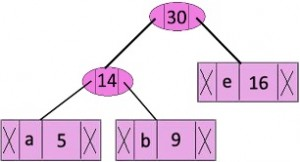Now min heap contains 3 nodes.

```character          Frequency
Internal Node         25
Internal Node         30
f               45 ```

Step 5: Extract two minimum frequency nodes. Add a new internal node with frequency 25 + 30 = 55Now min heap contains 2 nodes.

```character     Frequency
f         45
Internal Node    55```

Step 6: Extract two minimum frequency nodes. Add a new internal node with frequency 45 + 55 = 100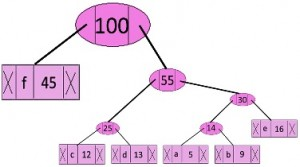Now min heap contains only one node.

```character      Frequency
Internal Node    100```

Since the heap contains only one node, the algorithm stops here.
Steps to print codes from Huffman Tree:
Traverse the tree formed starting from the root. Maintain an auxiliary array. While moving to the left child, write 0 to the array. While moving to the right child, write 1 to the array. Print the array when a leaf node is encountered.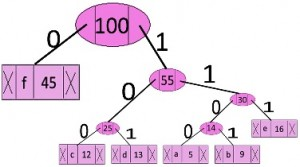The codes are as follows:

```character   code-word
f          0
c          100
d          101
a          1100
b          1101
e          111```

Below is the implementation of above approach:

## C

 `// C program for Huffman Coding``#include ``#include ` `// This constant can be avoided by explicitly``// calculating height of Huffman Tree``#define MAX_TREE_HT 100` `// A Huffman tree node``struct` `MinHeapNode {` `    ``// One of the input characters``    ``char` `data;` `    ``// Frequency of the character``    ``unsigned freq;` `    ``// Left and right child of this node``    ``struct` `MinHeapNode *left, *right;``};` `// A Min Heap:  Collection of``// min-heap (or Huffman tree) nodes``struct` `MinHeap {` `    ``// Current size of min heap``    ``unsigned size;` `    ``// capacity of min heap``    ``unsigned capacity;` `    ``// Array of minheap node pointers``    ``struct` `MinHeapNode** array;``};` `// A utility function allocate a new``// min heap node with given character``// and frequency of the character``struct` `MinHeapNode* newNode(``char` `data, unsigned freq)``{``    ``struct` `MinHeapNode* temp = (``struct` `MinHeapNode*)``malloc``(``        ``sizeof``(``struct` `MinHeapNode));` `    ``temp->left = temp->right = NULL;``    ``temp->data = data;``    ``temp->freq = freq;` `    ``return` `temp;``}` `// A utility function to create``// a min heap of given capacity``struct` `MinHeap* createMinHeap(unsigned capacity)` `{` `    ``struct` `MinHeap* minHeap``        ``= (``struct` `MinHeap*)``malloc``(``sizeof``(``struct` `MinHeap));` `    ``// current size is 0``    ``minHeap->size = 0;` `    ``minHeap->capacity = capacity;` `    ``minHeap->array = (``struct` `MinHeapNode**)``malloc``(``        ``minHeap->capacity * ``sizeof``(``struct` `MinHeapNode*));``    ``return` `minHeap;``}` `// A utility function to``// swap two min heap nodes``void` `swapMinHeapNode(``struct` `MinHeapNode** a,``                     ``struct` `MinHeapNode** b)` `{` `    ``struct` `MinHeapNode* t = *a;``    ``*a = *b;``    ``*b = t;``}` `// The standard minHeapify function.``void` `minHeapify(``struct` `MinHeap* minHeap, ``int` `idx)` `{` `    ``int` `smallest = idx;``    ``int` `left = 2 * idx + 1;``    ``int` `right = 2 * idx + 2;` `    ``if` `(left < minHeap->size``        ``&& minHeap->array[left]->freq``               ``< minHeap->array[smallest]->freq)``        ``smallest = left;` `    ``if` `(right < minHeap->size``        ``&& minHeap->array[right]->freq``               ``< minHeap->array[smallest]->freq)``        ``smallest = right;` `    ``if` `(smallest != idx) {``        ``swapMinHeapNode(&minHeap->array[smallest],``                        ``&minHeap->array[idx]);``        ``minHeapify(minHeap, smallest);``    ``}``}` `// A utility function to check``// if size of heap is 1 or not``int` `isSizeOne(``struct` `MinHeap* minHeap)``{` `    ``return` `(minHeap->size == 1);``}` `// A standard function to extract``// minimum value node from heap``struct` `MinHeapNode* extractMin(``struct` `MinHeap* minHeap)` `{` `    ``struct` `MinHeapNode* temp = minHeap->array;``    ``minHeap->array = minHeap->array[minHeap->size - 1];` `    ``--minHeap->size;``    ``minHeapify(minHeap, 0);` `    ``return` `temp;``}` `// A utility function to insert``// a new node to Min Heap``void` `insertMinHeap(``struct` `MinHeap* minHeap,``                   ``struct` `MinHeapNode* minHeapNode)` `{` `    ``++minHeap->size;``    ``int` `i = minHeap->size - 1;` `    ``while` `(i``           ``&& minHeapNode->freq``                  ``< minHeap->array[(i - 1) / 2]->freq) {` `        ``minHeap->array[i] = minHeap->array[(i - 1) / 2];``        ``i = (i - 1) / 2;``    ``}` `    ``minHeap->array[i] = minHeapNode;``}` `// A standard function to build min heap``void` `buildMinHeap(``struct` `MinHeap* minHeap)` `{` `    ``int` `n = minHeap->size - 1;``    ``int` `i;` `    ``for` `(i = (n - 1) / 2; i >= 0; --i)``        ``minHeapify(minHeap, i);``}` `// A utility function to print an array of size n``void` `printArr(``int` `arr[], ``int` `n)``{``    ``int` `i;``    ``for` `(i = 0; i < n; ++i)``        ``printf``(``"%d"``, arr[i]);` `    ``printf``(``"\n"``);``}` `// Utility function to check if this node is leaf``int` `isLeaf(``struct` `MinHeapNode* root)` `{` `    ``return` `!(root->left) && !(root->right);``}` `// Creates a min heap of capacity``// equal to size and inserts all character of``// data[] in min heap. Initially size of``// min heap is equal to capacity``struct` `MinHeap* createAndBuildMinHeap(``char` `data[],``                                      ``int` `freq[], ``int` `size)` `{` `    ``struct` `MinHeap* minHeap = createMinHeap(size);` `    ``for` `(``int` `i = 0; i < size; ++i)``        ``minHeap->array[i] = newNode(data[i], freq[i]);` `    ``minHeap->size = size;``    ``buildMinHeap(minHeap);` `    ``return` `minHeap;``}` `// The main function that builds Huffman tree``struct` `MinHeapNode* buildHuffmanTree(``char` `data[],``                                     ``int` `freq[], ``int` `size)` `{``    ``struct` `MinHeapNode *left, *right, *top;` `    ``// Step 1: Create a min heap of capacity``    ``// equal to size.  Initially, there are``    ``// modes equal to size.``    ``struct` `MinHeap* minHeap``        ``= createAndBuildMinHeap(data, freq, size);` `    ``// Iterate while size of heap doesn't become 1``    ``while` `(!isSizeOne(minHeap)) {` `        ``// Step 2: Extract the two minimum``        ``// freq items from min heap``        ``left = extractMin(minHeap);``        ``right = extractMin(minHeap);` `        ``// Step 3:  Create a new internal``        ``// node with frequency equal to the``        ``// sum of the two nodes frequencies.``        ``// Make the two extracted node as``        ``// left and right children of this new node.``        ``// Add this node to the min heap``        ``// '\$' is a special value for internal nodes, not``        ``// used``        ``top = newNode(``'\$'``, left->freq + right->freq);` `        ``top->left = left;``        ``top->right = right;` `        ``insertMinHeap(minHeap, top);``    ``}` `    ``// Step 4: The remaining node is the``    ``// root node and the tree is complete.``    ``return` `extractMin(minHeap);``}` `// Prints huffman codes from the root of Huffman Tree.``// It uses arr[] to store codes``void` `printCodes(``struct` `MinHeapNode* root, ``int` `arr[],``                ``int` `top)` `{` `    ``// Assign 0 to left edge and recur``    ``if` `(root->left) {` `        ``arr[top] = 0;``        ``printCodes(root->left, arr, top + 1);``    ``}` `    ``// Assign 1 to right edge and recur``    ``if` `(root->right) {` `        ``arr[top] = 1;``        ``printCodes(root->right, arr, top + 1);``    ``}` `    ``// If this is a leaf node, then``    ``// it contains one of the input``    ``// characters, print the character``    ``// and its code from arr[]``    ``if` `(isLeaf(root)) {` `        ``printf``(``"%c: "``, root->data);``        ``printArr(arr, top);``    ``}``}` `// The main function that builds a``// Huffman Tree and print codes by traversing``// the built Huffman Tree``void` `HuffmanCodes(``char` `data[], ``int` `freq[], ``int` `size)` `{``    ``// Construct Huffman Tree``    ``struct` `MinHeapNode* root``        ``= buildHuffmanTree(data, freq, size);` `    ``// Print Huffman codes using``    ``// the Huffman tree built above``    ``int` `arr[MAX_TREE_HT], top = 0;` `    ``printCodes(root, arr, top);``}` `// Driver code``int` `main()``{` `    ``char` `arr[] = { ``'a'``, ``'b'``, ``'c'``, ``'d'``, ``'e'``, ``'f'` `};``    ``int` `freq[] = { 5, 9, 12, 13, 16, 45 };` `    ``int` `size = ``sizeof``(arr) / ``sizeof``(arr);` `    ``HuffmanCodes(arr, freq, size);` `    ``return` `0;``}`

## C++

 `// C++ program for Huffman Coding``#include ``#include ``using` `namespace` `std;` `// This constant can be avoided by explicitly``// calculating height of Huffman Tree``#define MAX_TREE_HT 100` `// A Huffman tree node``struct` `MinHeapNode {` `    ``// One of the input characters``    ``char` `data;` `    ``// Frequency of the character``    ``unsigned freq;` `    ``// Left and right child of this node``    ``struct` `MinHeapNode *left, *right;``};` `// A Min Heap: Collection of``// min-heap (or Huffman tree) nodes``struct` `MinHeap {` `    ``// Current size of min heap``    ``unsigned size;` `    ``// capacity of min heap``    ``unsigned capacity;` `    ``// Attay of minheap node pointers``    ``struct` `MinHeapNode** array;``};` `// A utility function allocate a new``// min heap node with given character``// and frequency of the character``struct` `MinHeapNode* newNode(``char` `data, unsigned freq)``{``    ``struct` `MinHeapNode* temp``        ``= (``struct` `MinHeapNode*)``malloc``(``sizeof``(``struct` `MinHeapNode));` `    ``temp->left = temp->right = NULL;``    ``temp->data = data;``    ``temp->freq = freq;` `    ``return` `temp;``}` `// A utility function to create``// a min heap of given capacity``struct` `MinHeap* createMinHeap(unsigned capacity)` `{` `    ``struct` `MinHeap* minHeap``        ``= (``struct` `MinHeap*)``malloc``(``sizeof``(``struct` `MinHeap));` `    ``// current size is 0``    ``minHeap->size = 0;` `    ``minHeap->capacity = capacity;` `    ``minHeap->array``        ``= (``struct` `MinHeapNode**)``malloc``(minHeap->``capacity * ``sizeof``(``struct` `MinHeapNode*));``    ``return` `minHeap;``}` `// A utility function to``// swap two min heap nodes``void` `swapMinHeapNode(``struct` `MinHeapNode** a,``                    ``struct` `MinHeapNode** b)` `{` `    ``struct` `MinHeapNode* t = *a;``    ``*a = *b;``    ``*b = t;``}` `// The standard minHeapify function.``void` `minHeapify(``struct` `MinHeap* minHeap, ``int` `idx)` `{` `    ``int` `smallest = idx;``    ``int` `left = 2 * idx + 1;``    ``int` `right = 2 * idx + 2;` `    ``if` `(left < minHeap->size && minHeap->array[left]->``freq < minHeap->array[smallest]->freq)``        ``smallest = left;` `    ``if` `(right < minHeap->size && minHeap->array[right]->``freq < minHeap->array[smallest]->freq)``        ``smallest = right;` `    ``if` `(smallest != idx) {``        ``swapMinHeapNode(&minHeap->array[smallest],``                        ``&minHeap->array[idx]);``        ``minHeapify(minHeap, smallest);``    ``}``}` `// A utility function to check``// if size of heap is 1 or not``int` `isSizeOne(``struct` `MinHeap* minHeap)``{` `    ``return` `(minHeap->size == 1);``}` `// A standard function to extract``// minimum value node from heap``struct` `MinHeapNode* extractMin(``struct` `MinHeap* minHeap)` `{` `    ``struct` `MinHeapNode* temp = minHeap->array;``    ``minHeap->array``        ``= minHeap->array[minHeap->size - 1];` `    ``--minHeap->size;``    ``minHeapify(minHeap, 0);` `    ``return` `temp;``}` `// A utility function to insert``// a new node to Min Heap``void` `insertMinHeap(``struct` `MinHeap* minHeap,``                ``struct` `MinHeapNode* minHeapNode)` `{` `    ``++minHeap->size;``    ``int` `i = minHeap->size - 1;` `    ``while` `(i && minHeapNode->freq < minHeap->array[(i - 1) / 2]->freq) {` `        ``minHeap->array[i] = minHeap->array[(i - 1) / 2];``        ``i = (i - 1) / 2;``    ``}` `    ``minHeap->array[i] = minHeapNode;``}` `// A standard function to build min heap``void` `buildMinHeap(``struct` `MinHeap* minHeap)` `{` `    ``int` `n = minHeap->size - 1;``    ``int` `i;` `    ``for` `(i = (n - 1) / 2; i >= 0; --i)``        ``minHeapify(minHeap, i);``}` `// A utility function to print an array of size n``void` `printArr(``int` `arr[], ``int` `n)``{``    ``int` `i;``    ``for` `(i = 0; i < n; ++i)``        ``cout<< arr[i];` `    ``cout<<``"\n"``;``}` `// Utility function to check if this node is leaf``int` `isLeaf(``struct` `MinHeapNode* root)` `{` `    ``return` `!(root->left) && !(root->right);``}` `// Creates a min heap of capacity``// equal to size and inserts all character of``// data[] in min heap. Initially size of``// min heap is equal to capacity``struct` `MinHeap* createAndBuildMinHeap(``char` `data[], ``int` `freq[], ``int` `size)` `{` `    ``struct` `MinHeap* minHeap = createMinHeap(size);` `    ``for` `(``int` `i = 0; i < size; ++i)``        ``minHeap->array[i] = newNode(data[i], freq[i]);` `    ``minHeap->size = size;``    ``buildMinHeap(minHeap);` `    ``return` `minHeap;``}` `// The main function that builds Huffman tree``struct` `MinHeapNode* buildHuffmanTree(``char` `data[], ``int` `freq[], ``int` `size)` `{``    ``struct` `MinHeapNode *left, *right, *top;` `    ``// Step 1: Create a min heap of capacity``    ``// equal to size. Initially, there are``    ``// modes equal to size.``    ``struct` `MinHeap* minHeap = createAndBuildMinHeap(data, freq, size);` `    ``// Iterate while size of heap doesn't become 1``    ``while` `(!isSizeOne(minHeap)) {` `        ``// Step 2: Extract the two minimum``        ``// freq items from min heap``        ``left = extractMin(minHeap);``        ``right = extractMin(minHeap);` `        ``// Step 3: Create a new internal``        ``// node with frequency equal to the``        ``// sum of the two nodes frequencies.``        ``// Make the two extracted node as``        ``// left and right children of this new node.``        ``// Add this node to the min heap``        ``// '\$' is a special value for internal nodes, not used``        ``top = newNode(``'\$'``, left->freq + right->freq);` `        ``top->left = left;``        ``top->right = right;` `        ``insertMinHeap(minHeap, top);``    ``}` `    ``// Step 4: The remaining node is the``    ``// root node and the tree is complete.``    ``return` `extractMin(minHeap);``}` `// Prints huffman codes from the root of Huffman Tree.``// It uses arr[] to store codes``void` `printCodes(``struct` `MinHeapNode* root, ``int` `arr[], ``int` `top)` `{` `    ``// Assign 0 to left edge and recur``    ``if` `(root->left) {` `        ``arr[top] = 0;``        ``printCodes(root->left, arr, top + 1);``    ``}` `    ``// Assign 1 to right edge and recur``    ``if` `(root->right) {` `        ``arr[top] = 1;``        ``printCodes(root->right, arr, top + 1);``    ``}` `    ``// If this is a leaf node, then``    ``// it contains one of the input``    ``// characters, print the character``    ``// and its code from arr[]``    ``if` `(isLeaf(root)) {` `        ``cout<< root->data <<``": "``;``        ``printArr(arr, top);``    ``}``}` `// The main function that builds a``// Huffman Tree and print codes by traversing``// the built Huffman Tree``void` `HuffmanCodes(``char` `data[], ``int` `freq[], ``int` `size)` `{``    ``// Construct Huffman Tree``    ``struct` `MinHeapNode* root``        ``= buildHuffmanTree(data, freq, size);` `    ``// Print Huffman codes using``    ``// the Huffman tree built above``    ``int` `arr[MAX_TREE_HT], top = 0;` `    ``printCodes(root, arr, top);``}` `// Driver code``int` `main()``{` `    ``char` `arr[] = { ``'a'``, ``'b'``, ``'c'``, ``'d'``, ``'e'``, ``'f'` `};``    ``int` `freq[] = { 5, 9, 12, 13, 16, 45 };` `    ``int` `size = ``sizeof``(arr) / ``sizeof``(arr);` `    ``HuffmanCodes(arr, freq, size);` `    ``return` `0;``}`

## C++

 `// C++ program for Huffman Coding with STL``#include ``using` `namespace` `std;` `// A Huffman tree node``struct` `MinHeapNode {` `    ``// One of the input characters``    ``char` `data;` `    ``// Frequency of the character``    ``unsigned freq;` `    ``// Left and right child``    ``MinHeapNode *left, *right;` `    ``MinHeapNode(``char` `data, unsigned freq)` `    ``{` `        ``left = right = NULL;``        ``this``->data = data;``        ``this``->freq = freq;``    ``}``};` `// For comparison of``// two heap nodes (needed in min heap)``struct` `compare {` `    ``bool` `operator()(MinHeapNode* l, MinHeapNode* r)` `    ``{``        ``return` `(l->freq > r->freq);``    ``}``};` `// Prints huffman codes from``// the root of Huffman Tree.``void` `printCodes(``struct` `MinHeapNode* root, string str)``{` `    ``if` `(!root)``        ``return``;` `    ``if` `(root->data != ``'\$'``)``        ``cout << root->data << ``": "` `<< str << ``"\n"``;` `    ``printCodes(root->left, str + ``"0"``);``    ``printCodes(root->right, str + ``"1"``);``}` `// The main function that builds a Huffman Tree and``// print codes by traversing the built Huffman Tree``void` `HuffmanCodes(``char` `data[], ``int` `freq[], ``int` `size)``{``    ``struct` `MinHeapNode *left, *right, *top;` `    ``// Create a min heap & inserts all characters of data[]``    ``priority_queue, compare> minHeap;` `    ``for` `(``int` `i = 0; i < size; ++i)``        ``minHeap.push(``new` `MinHeapNode(data[i], freq[i]));` `    ``// Iterate while size of heap doesn't become 1``    ``while` `(minHeap.size() != 1) {` `        ``// Extract the two minimum``        ``// freq items from min heap``        ``left = minHeap.top();``        ``minHeap.pop();` `        ``right = minHeap.top();``        ``minHeap.pop();` `        ``// Create a new internal node with``        ``// frequency equal to the sum of the``        ``// two nodes frequencies. Make the``        ``// two extracted node as left and right children``        ``// of this new node. Add this node``        ``// to the min heap '\$' is a special value``        ``// for internal nodes, not used``        ``top = ``new` `MinHeapNode(``'\$'``, left->freq + right->freq);` `        ``top->left = left;``        ``top->right = right;` `        ``minHeap.push(top);``    ``}` `    ``// Print Huffman codes using``    ``// the Huffman tree built above``    ``printCodes(minHeap.top(), ``""``);``}` `// Driver Code``int` `main()``{` `    ``char` `arr[] = { ``'a'``, ``'b'``, ``'c'``, ``'d'``, ``'e'``, ``'f'` `};``    ``int` `freq[] = { 5, 9, 12, 13, 16, 45 };` `    ``int` `size = ``sizeof``(arr) / ``sizeof``(arr);` `    ``HuffmanCodes(arr, freq, size);` `    ``return` `0;``}` `// This code is contributed by Aditya Goel`

## Java

 `import` `java.util.PriorityQueue;``import` `java.util.Scanner;``import` `java.util.Comparator;` `// node class is the basic structure``// of each node present in the Huffman - tree.``class` `HuffmanNode {` `    ``int` `data;``    ``char` `c;` `    ``HuffmanNode left;``    ``HuffmanNode right;``}` `// comparator class helps to compare the node``// on the basis of one of its attribute.``// Here we will be compared``// on the basis of data values of the nodes.``class` `MyComparator ``implements` `Comparator {``    ``public` `int` `compare(HuffmanNode x, HuffmanNode y)``    ``{` `        ``return` `x.data - y.data;``    ``}``}` `public` `class` `Huffman {` `    ``// recursive function to print the``    ``// huffman-code through the tree traversal.``    ``// Here s is the huffman - code generated.``    ``public` `static` `void` `printCode(HuffmanNode root, String s)``    ``{` `        ``// base case; if the left and right are null``        ``// then its a leaf node and we print``        ``// the code s generated by traversing the tree.``        ``if` `(root.left``                ``== ``null``            ``&& root.right``                   ``== ``null``            ``&& Character.isLetter(root.c)) {` `            ``// c is the character in the node``            ``System.out.println(root.c + ``":"` `+ s);` `            ``return``;``        ``}` `        ``// if we go to left then add "0" to the code.``        ``// if we go to the right add"1" to the code.` `        ``// recursive calls for left and``        ``// right sub-tree of the generated tree.``        ``printCode(root.left, s + ``"0"``);``        ``printCode(root.right, s + ``"1"``);``    ``}` `    ``// main function``    ``public` `static` `void` `main(String[] args)``    ``{` `        ``Scanner s = ``new` `Scanner(System.in);` `        ``// number of characters.``        ``int` `n = ``6``;``        ``char``[] charArray = { ``'a'``, ``'b'``, ``'c'``, ``'d'``, ``'e'``, ``'f'` `};``        ``int``[] charfreq = { ``5``, ``9``, ``12``, ``13``, ``16``, ``45` `};` `        ``// creating a priority queue q.``        ``// makes a min-priority queue(min-heap).``        ``PriorityQueue q``            ``= ``new` `PriorityQueue(n, ``new` `MyComparator());` `        ``for` `(``int` `i = ``0``; i < n; i++) {` `            ``// creating a Huffman node object``            ``// and add it to the priority queue.``            ``HuffmanNode hn = ``new` `HuffmanNode();` `            ``hn.c = charArray[i];``            ``hn.data = charfreq[i];` `            ``hn.left = ``null``;``            ``hn.right = ``null``;` `            ``// add functions adds``            ``// the huffman node to the queue.``            ``q.add(hn);``        ``}` `        ``// create a root node``        ``HuffmanNode root = ``null``;` `        ``// Here we will extract the two minimum value``        ``// from the heap each time until``        ``// its size reduces to 1, extract until``        ``// all the nodes are extracted.``        ``while` `(q.size() > ``1``) {` `            ``// first min extract.``            ``HuffmanNode x = q.peek();``            ``q.poll();` `            ``// second min extarct.``            ``HuffmanNode y = q.peek();``            ``q.poll();` `            ``// new node f which is equal``            ``HuffmanNode f = ``new` `HuffmanNode();` `            ``// to the sum of the frequency of the two nodes``            ``// assigning values to the f node.``            ``f.data = x.data + y.data;``            ``f.c = ``'-'``;` `            ``// first extracted node as left child.``            ``f.left = x;` `            ``// second extracted node as the right child.``            ``f.right = y;` `            ``// marking the f node as the root node.``            ``root = f;` `            ``// add this node to the priority-queue.``            ``q.add(f);``        ``}` `        ``// print the codes by traversing the tree``        ``printCode(root, ``""``);``    ``}``}` `// This code is contributed by Kunwar Desh Deepak Singh`

## Python3

 `# A Huffman Tree Node``class` `node:``    ``def` `__init__(``self``, freq, symbol, left``=``None``, right``=``None``):``        ``# frequency of symbol``        ``self``.freq ``=` `freq` `        ``# symbol name (charecter)``        ``self``.symbol ``=` `symbol` `        ``# node left of current node``        ``self``.left ``=` `left` `        ``# node right of current node``        ``self``.right ``=` `right` `        ``# tree direction (0/1)``        ``self``.huff ``=` `''` `# utility function to print huffman``# codes for all symbols in the newly``# created Huffman tree`  `def` `printNodes(node, val``=``''):``    ``# huffman code for current node``    ``newVal ``=` `val ``+` `str``(node.huff)` `    ``# if node is not an edge node``    ``# then traverse inside it``    ``if``(node.left):``        ``printNodes(node.left, newVal)``    ``if``(node.right):``        ``printNodes(node.right, newVal)` `        ``# if node is edge node then``        ``# display its huffman code``    ``if``(``not` `node.left ``and` `not` `node.right):``        ``print``(f``"{node.symbol} -> {newVal}"``)`  `# charecters for huffman tree``chars ``=` `[``'a'``, ``'b'``, ``'c'``, ``'d'``, ``'e'``, ``'f'``]` `# frequency of charecters``freq ``=` `[ ``5``, ``9``, ``12``, ``13``, ``16``, ``45``]` `# list containing unused nodes``nodes ``=` `[]` `# converting ccharecters and frequencies``# into huffman tree nodes``for` `x ``in` `range``(``len``(chars)):``    ``nodes.append(node(freq[x], chars[x]))` `while` `len``(nodes) > ``1``:``    ``# sort all the nodes in ascending order``    ``# based on theri frequency``    ``nodes ``=` `sorted``(nodes, key``=``lambda` `x: x.freq)` `    ``# pick 2 smallest nodes``    ``left ``=` `nodes[``0``]``    ``right ``=` `nodes[``1``]` `    ``# assign directional value to these nodes``    ``left.huff ``=` `0``    ``right.huff ``=` `1` `    ``# combine the 2 smallest nodes to create``    ``# new node as their parent``    ``newNode ``=` `node(left.freq``+``right.freq, left.symbol``+``right.symbol, left, right)` `    ``# remove the 2 nodes and add their``    ``# parent as new node among others``    ``nodes.remove(left)``    ``nodes.remove(right)``    ``nodes.append(newNode)` `# Huffman Tree is ready!``printNodes(nodes[``0``])`

Output:

```f: 0
c: 100
d: 101
a: 1100
b: 1101
e: 111```

Time complexity: O(nlogn) where n is the number of unique characters. If there are n nodes, extractMin() is called 2*(n – 1) times. extractMin() takes O(logn) time as it calles minHeapify(). So, overall complexity is O(nlogn).
If the input array is sorted, there exists a linear time algorithm. We will soon be discussing in our next post.

#### Applications of Huffman Coding:

1. They are used for transmitting fax and text.
2. They are used by conventional compression formats like PKZIP, GZIP, etc.

It is useful in cases where there is a series of frequently occurring characters.

Reference:
http://en.wikipedia.org/wiki/Huffman_coding